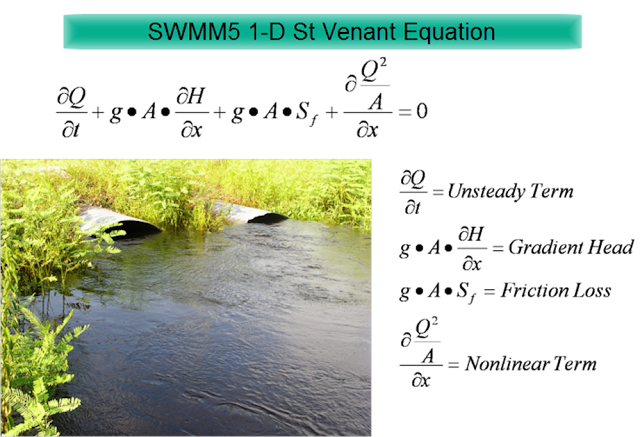All ForumsOverview

In this blog we example the St Venant terms used in SWMM5 as equations, table, graphs and units.  We use a QA/QC version of SWMM 5 that lists many more link, node, system and Subcatchment variables than the default SWMM 5 GUI and engine.  This also applies to #InfoSWMM and any software the uses the #SWMM5 engine.

How are the St Venant Terms used in SWMM5?

Figure 1 shows the terms and Figure 2  and Figure 3 shows the terms in a SWMM5 table and SWMM5 graph.

dq2 = Time Step * Area wtd * (Head Downstream – Head Upstream) / Link Length or

dq2 = Time Step * Area wtd * (HGL) / Link Length Qnew = (Qold – dq2 + dq3 + dq4) / ( 1 + dq1) when the force main is full dq3 and dq4 are zero and

Qnew = (Qold – dq2) / ( 1 + dq1) The dq4 term in dynamic.c uses the area upstream (a1) and area downstream (a2), the midpoint velocity, the sigma factor (a function of the link Froude number), the link length and the time step or

dq4 = Time Step * Velocity * Velocity * (a2 – a1) / Link Length * Sigma the dq3 term in dynamic.c uses the current midpoint area (a function of the midpoint depth), the sigma factor and the midpoint velocity

dq3 = 2 * Velocity * ( Amid(current iteration) – Amid (last time step) * Sigma

dq1 = Time Step * RoughFactor / Rwtd^1.333 * |Velocity| The weighted area (Awtd) is used in the dq2 term of the St. Venant equation:

dq2 = Time Step * Awtd * (Head Downstream – Head Upstream) / Link Length

You can also see the QA/QC report for SWMM 5 https://www.epa.gov/water-research/storm-water-management-model-swmm#downloads

How are the St Venant Units used in #SWMM5?

The new flow (Q) calculated at during each iteration of time step as

(1) Q for the new iteration = (Q at the Old Time Step – DQ2 + DQ3 + DQ4 ) / ( 1.0 + DQ1 + DQ5)

In which DQ2, DQ3 and DQ4 all have units of flow (note internally SWMM 5 has units of CFS and the flows are converted to the user units in the output file, graphs and tables of SWMM 5).

The equations and units for DQ2, DQ3 and DQ4 are:

(2) Units of DQ2 = DT * GRAVITY * aWtd * ( H2 – H1) / Length = second * feet/second^2 * feet^2 * feet / feet = feet^3/second = CFS

(3) Units of DQ3 = 2 * Velocity * ( aMid – aOld) * Sigma = feet/second * feet^2 = feet^3/second = CFS

(4) Units of DQ4 = DT * Velocity * Velocity * ( aMid – aOld) * Sigma / Length = second * feet/second * feet/second * feet^2 / feet = feet^3/second = CFS

The equations and units for DQ1 and DQ5 are:

(5) Units of DQ1 = DT * GRAVITY * (n/PHI)^2 * Velocity / Hydraulic Radius^1.333 = second * feet/second^2 * second^2 * feet^1/3 * feet/second / feet^1.33 = Dimensionless

(6) Units of DQ5 = K * Q / Area / 2 / Length * DT = feet^3/second * 1/feet^2 * 1/feet * second = DimensionlessFigure 1.  St Venant Terms in Table and Graphs for #SWMM5 for dq1, dq2, dq3, dq4, dq5, dq6Figure 2.  St Venant Equation in SWMM5

You need to be a member of SWMM 5 or SWMM or EPASWMM - (not formally associated with EPA) to add comments!